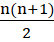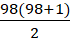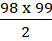# CAT 2019 Question Paper | Quants Slot 2

###### CAT Previous Year Paper | CAT Quants Questions | Question 29

The best way to go about preparing for the CAT exam is to practice CAT previous year paper questions. They help you understand the pattern and the difficulty level of the questions that you'll face in the CAT Question Paper. Do not stretch yourself to do extremely difficult questions for acing your CAT preparation online, however, it'll be naive of you if you do not practice the questions from CAT previous year paper. Start right away with this question from Progressions taken from CAT 2019 Question Paper.
If you are looking for more questions to practice, visit 2IIM's CAT Question Bank.

Question 29 : If (2n+1) + (2n+3) + (2n+5) + ... + (2n+47) = 5280 , then what is the value of 1+2+3+ ... +n ? [TITA]

## Best CAT Coaching in Chennai

#### CAT Coaching in Chennai - CAT 2022Limited Seats Available - Register Now!

Given series - (2n+1) + (2n+3) + (2n+5) + ... + (2n+47) = 5280
Isolate 2n terms on one side
(2n + 2n +.... + 2n) + (1 +3 + 5 +.... + 47) = 5280
Odd numbers from 1 to 47 are added in the above series.
Number of terms from 1 to 47 = 24 terms
Therefore, the number of 2n terms = 24
For computing the value of (1 +3 + 5 +.... + 47),
We know 1 = 12, 1 +3 = 22, 1 +3 +5 = 32 and so on
So, (1 +3 + 5 +.... + 47) = 242
So, 2n x 24 + 242 = 5280
2n x 24 = 5280 - 242
2n = 220 - 24
n = 110 - 12
n = 98
So, value of 1+2+3+...+98 ===Value of 1+2+3+...+98 = 4851

The question is "If (2n+1) + (2n+3) + (2n+5) + ... + (2n+47) = 5280 , then what is the value of 1+2+3+ ... +n ? [TITA] "

##### Hence, the answer is 4851

###### CAT Coaching in ChennaiCAT 2023

Classroom Batches Starting Now! @Gopalapuram

###### Best CAT Coaching in Chennai Introductory offer of 5000/-

Attend a Demo Class

##### Where is 2IIM located?

2IIM Online CAT Coaching
A Fermat Education Initiative,
58/16, Indira Gandhi Street,
Kaveri Rangan Nagar, Saligramam, Chennai 600 093

##### How to reach 2IIM?

Mobile: (91) 99626 48484 / 94459 38484
WhatsApp: WhatsApp Now
Email: info@2iim.com